Trial ends in
JoVE Science Education
Mechanical Engineering

A subscription to JoVE is required to view this content.
You will only be able to see the first 20 seconds.

Heat Exchanger Analysis

# Heat Exchanger Analysis

### Overview

Source: Alexander S Rattner and Christopher J Greer; Department of Mechanical and Nuclear Engineering, The Pennsylvania State University, University Park, PA

Heat exchangers transfer thermal energy between two fluid streams, and are ubiquitous in energy systems. Common applications include car radiators (heat transfer from hot engine coolant to surrounding air), refrigerator evaporators (air inside refrigerator compartment to evaporating refrigerant), and cooling towers in power plants (condensing steam to evaporating water and ambient air). The objective of this experiment is to introduce experimental measurement (rating) and modeling procedures for heat exchangers.

In this experiment, a water-to-water tube-in-tube heat exchanger will be constructed, and evaluated. Temperature and flow rate measurements will be employed to determine the heat transfer rate (Q) and overall conductance (UA). The measured heat exchanger UA will be compared with predicted values for the geometry and operating conditions.

### Principles

In a heat exchanger (HX), thermal energy is transferred from a hot (H) fluid stream to a cold (C) fluid stream. Each stream may have a different mass flow rate (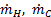) and specific heat (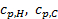). As the streams pass through a HX, the temperature of the hot flow decreases, and the temperature of the cold stream increases. During steady operation, if heat leakage to the surroundings is negligible, then the energy changes of the two streams from inlets to outlets must balance. This energy change is the heat exchanger heat transfer rate Q.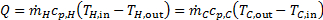(1)

In this experiment, heat transfer performance is analyzed for a counter-flow tube-in-tube heat exchanger. Here hot fluid flows in one direction through an inner tube. Cold fluid flows in the opposite direction through the annular space between the inner tube and an outer tube. The average temperature difference that drives heat transfer between the two streams is the log-mean temperature difference (LMTD, Fig. 1), defined in Eqn. 2 for the counter-flow HX configuration. If the temperature differences at both ends of the heat exchanger match within measurement precision (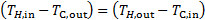), a simpler LMTD formula should be used.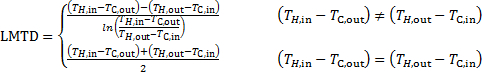(2)

The heat transfer capacity of a heat exchanger is measured in terms of the overall conductance (UA). This quantity has units of W K-1 (heat transfer rate per temperature difference). The UA can be evaluated from measured heat transfer rates and fluid temperatures: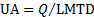(3)

The tube-in-tube HX geometry is defined by the length of the tubes (L), the inner tube inner and outer diameters (IDi, ODi), and outer tube diameters (IDo, ODo). Using these parameters and material properties, the heat exchanger UA can be predicted by accounting for the thermal resistances between the two streams. For fully developed laminar flow in the inner tube, the thermal resistance from the inner stream to the inner tube inner wall is: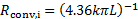where k is the fluid thermal conductivity (0.61 W m-1 K-1 for water). The thermal resistance for conduction through the inner tube wall, is: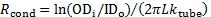(ktube = 160 W m-1 K-1 for aluminum). Finally, for fully developed laminar flow in a narrow annulus, the convection resistance from the outside of the inner tube to the outer stream is: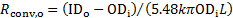. Under these conditions, the predicted HX UA is: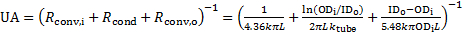(4)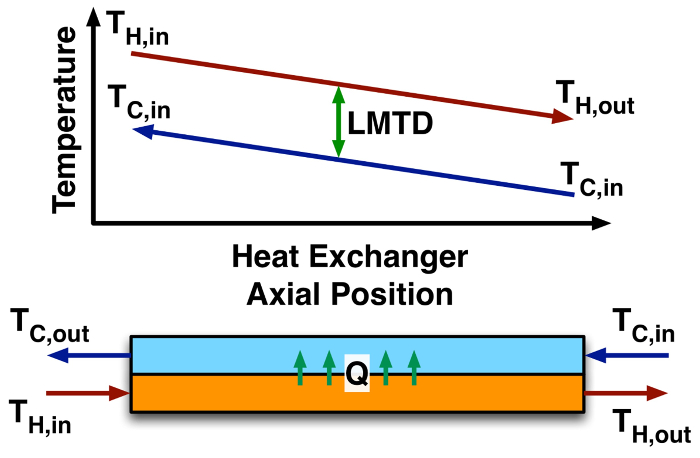Figure 1: Cold and hot stream temperature profiles and log-mean temperature difference in a counter-flow heat exchanger.

### Procedure

1. Fabrication of heat exchanger system (see schematic and photograph, Fig. 2)

1. Affix two plastic water reservoirs (~1 liter each) to a work surface (~0.6 m apart). If these are covered containers, drill holes in the lid for the inlet and outlet water lines and pump power cable. These will serve as the hot and cold water reservoirs.
2. Mount one small submersible pump in each reservoir.
3. Vertically mount two water flow meters (rotameters), one near each reservoir. Use soft PVC tubing to connect the flow meter inlets to the pump discharge ports.
4. Install the heat exchanger (HX) outer tube (~0.3 m long, outer diameter OD = 12.7 mm, inner diameter ID = 9.5 mm) into two compression pipe tee fittings (see Fig. 2). Connect a flexible PVC tube (OD = 12.7 mm, ID = 6.2 mm) from the side port on one tee fitting to the hot flow meter outlet.
5. Cut an aluminium tube (OD = 7.9 mm, ID = 6.2 mm) to the length of the heat exchanger, including the tee fittings on the end (~0.38 m long), and insert it into the heat exchanger assembly. The aluminium tube should slide snugly into the soft connecting PVC tube (OD = 12.7 mm, ID = 6.2 mm) at the end of the compression fitting.
6. Connect a soft PVC tube from the compression fitting at the other end of the HX assembly to the hot water reservoir. Tighten the compression fittings to seal the soft plastic tubing around the aluminium tube. This will separate the hot flow through the inner aluminium tube from the outer cold flow.
7. Connect a flexible PVC tube from the side port on one tee fitting to the cold flow meter outlet. Connect a PVC tube from the side port on the other tee fitting to the cold water reservoir (return flow). The hot and cold stream inlets to the HX should be on opposite ends.
8. Drill small holes (~1.6 mm diameter) through one side of the soft plastic tubes near each heat exchanger inlet and outlet port (4 total). Gently insert a thermocouple probe into each port so that the probe tip is approximately in the center of the tube. Connect the thermocouple probes to a thermocouple reader
9. Using epoxy or similar adhesive to seal the small gap in the tubes around the thermocouple probes so that no water leaks out.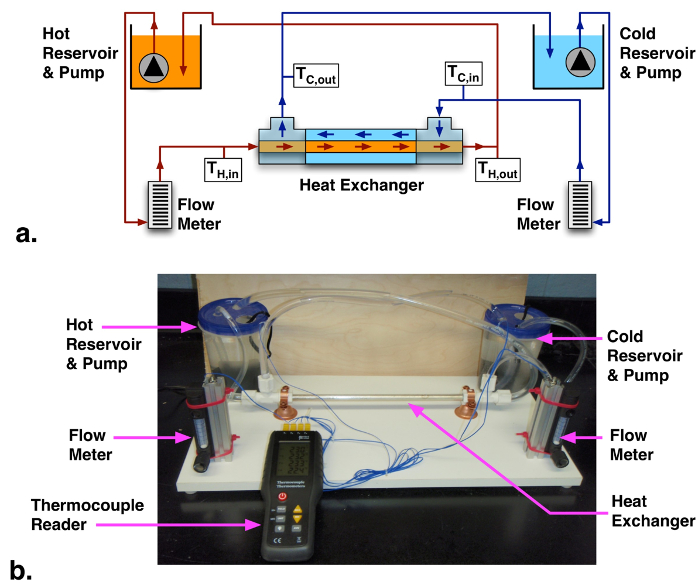Figure 2: (a) Schematic and (b) labelled photograph of heat exchanger rating experimental system

2. Operation

1. Fill the cold reservoir with room temperature tap water, and the hot reservoir with warm water.
2. Turn on the two water pumps, and use the flow meter needle valves to adjust the flow rates to desired values (e.g., 0.1 l min-1). It may be necessary to circulate water at a higher flow rate initially to clear out trapped air bubbles.
3. Allow the system to stabilize for a couple minutes, and then record the four thermocouple measurements representing the inlet and outlet temperatures. Record a few sets of readings for each flow condition. If available, the hold function on the thermocouple reader can freeze readings to help recording.
4. Collect temperature measurements at a few sets of hot and cold water flow rates. Periodically refill the reservoirs with fresh hot and cold water to maintain sufficient average temperature differences (~5 - 10°C).

3. Analysis

1. For each condition, compare the hot- and cold-stream energy change rates (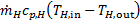,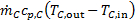). For water, cp = 4.2 kJ kg-1 K-1. and the volume flow rate can be multiplied by density (ρwater = 997 kg m-3) to find the mass flow rate. Do the energy change rates (Q) match, as assumed in Eqn. 1?
2. Evaluate the LMTD for each condition following Eqn. 2 using Q from Step 3.1. Evaluate the heat exchanger UA (defined in Eqn. 3). Is this quantity approximately constant for considered conditions?
3. Evaluate the theoretical UA for fully developed laminar flow in this HX (Eqn. 4) using the mean heat transfer rate ((QC+QH)/2). How does this theoretical value compare to the measured value?

Heat exchangers are ubiquitous components in energy systems. Some common examples are car radiators and refrigerator evaporators. In both cases, the heat exchanger is facilitating the transfer of heat, from one stream of fluid to another. From these examples, it is clear that heat exchangers are important in a variety of systems; Primarily providing thermal management or transitions in thermodynamic cycles. An understanding of how to model and rate heat exchangers is important for optimizing designs and integrating heat exchangers into larger systems. This video will illustrate some principles of heat exchanger design and analysis, and then demonstrate those concepts on a simple tube-in-tube heat exchanger design. At the end, some common applications will be explored.

A well designed heat exchanger should facilitate efficient, continuous heat transfer between two fluid streams, without allowing them to mix. As two fluid streams enter a heat exchanger, they are brought into close thermal contact across a physical barrier. Heat exchange is driven by a local temperature differences as the fluids progress, until the fluids reach the exit. The result is that the hotter of the two fluids entering the heat exchanger will exit with a reduced temperature, and the colder of the two with an increased temperature. During steady operation, the heat transfer rate of the hot fluid is determined by the mass flow rate and specific heat of the fluid, multiplied by the difference in temperature between the entrance and the exit. The same formula applies to the cold fluid when the corresponding values are used. If heat leakage to the surroundings is negligible, the magnitude of the two transfer rates will be equal. This means that any heat lost by the hot fluid, is gained by the cold fluid. The overall conductance in Watts per Kelvin, is a measure of the heat transfer capacity of a heat exchanger. Let's analyze a particular geometry known as a counter-flow, tube-in-tube heat exchanger. In this design, the hot fluid flows in one direction inside a straight section of tube. The cold fluid flows in the opposite direction, in the annulus between the hot tube and a second outer tube. The average temperature difference driving heat transfer between the two streams in this geometry, is the log mean temperature difference, which can be calculated from the input and output temperatures of both streams. Using this model of heat exchanger operation, we can answer two different types of heat exchanger analysis problems. Rating and design. If the heat transfer rate and the log mean temperature difference are known, for example by experimental measurement, then the overall conductance can be calculated from the ratio of the two. For the purpose of design however, it is useful to predict what the overall conductance will be from the geometry and material properties of the heat exchanger. This can be done by finding the sum of thermal resistances between the two streams. For the tube-in-tube geometry, these resistances are determined by: convection in the hot fluid tube, conduction through the inner tube wall, and convection again in the cold fluid annulus. The inverse of this sum gives the overall conductance for the tube-in-tube counter-flow heat exchanger. Now that we have seen how to analyze the heat exchanger, let's test one in the laboratory.

Affix two plastic containers on opposite sides of the work surface to serve as hot and cold water reservoirs. If necessary, drill holes in the lids for inlet and outlet water lines, as well as the pump power cable. When you are finished, mount a small, submersible pump in each reservoir. Next, mount a water flow meter, or rotameter, vertically near each reservoir, and then use soft PVC tubing to connect the pump outlets to the flow meter inlets. Now, install two compression pipe tee fittings onto the outer cold water tube of the heat exchanger. Connect one flexible PVC tube, from the side port of the nearby tee fitting, to the outlet of hot flow meter. For the hot counter flow tube, cut a section of aluminum tubing equal in length to the heat exchanger, including the tee fittings on the end, and insert it into the assembly. Next, connect a soft plastic tube, from the compression fitting at other end of the heat exchanger assembly, to the hot water reservoir. Tighten the compression fittings to seal the soft plastic tubing around the aluminum tube. This will separate the hot flow, through the inner aluminum tube, from the outer cold flow. Connect the flexible PVC tube, from the side port on one tee fitting, to the cold flow meter outlet, and then connect a second tube to the side port of the other tee fitting returning to the cold water reservoir. Before continuing, confirm that the hot and cold stream inlets, to the heat exchanger, are on opposite ends. Drill a small hole through one side of the soft plastic tube near each inlet and outlet port of the heat exchanger. Gently insert a thermocouple probe into each port so that the probe tip is approximately in the center of the tube. Finally, use the epoxy, or similar adhesive, to seal the small gap in the tubes around the thermocouple probes against water leaks. When the epoxy is cured, connect all four thermocouple probes to a thermocouple reader. Now that the assembly is complete, you are ready to begin testing.

Fill the cold reservoir with room temperature tap water, and the hot reservoir with warm water. Turn on both water pumps and adjust the needle valves on the flow meters to increase the flow in both loops. Allow the water to circulate long enough to flush out any trapped air bubbles. Once the air bubbles are removed, adjust the flow rate in both loops to approximately 0.1 liters per minute. Wait a few minutes to allow the system to stabilize, and then record the inlet and outlet temperatures reported by the four thermocouple readings. Your thermocouple reader may have a hold function to freeze the current values while you are recording. Record five more sets of readings at these flow conditions. Repeat these measurements for flow rates of approximately 0.125 liters per minute, and 0.15 liters per minute. If the temperature difference between the hot and cold inputs drops below 5 degrees Celsius, refill the reservoirs with fresh hot and cold water. Now that the measurements are complete, let's take a look at the results.

You should have 18 sets of data, and for each set a measured volume flow rate. Note for these tests, the same flow rate, V-dot, is used for the hot and cold streams. First, use the density of water to convert each value of the volume flow rate to a mass flow rate. Now, calculate the rate of energy change for the hot and cold streams in each set by multiplying the mass flow rate, the specific heat of water, and the respective temperature differences. In the principle section, we assumed that the magnitude of these rates would be equal. Propagate uncertainties so that you can compare them. In most cases, the heat transfer rates match to within uncertainty; However, as the flow rate decreases, there is a trend towards increased heat loss from the hot fluid, as compared to the heat gained by the cold fluid. This is likely the result of heat loss to the surrounding environment; But since the effect is small, the average heat transfer rate can be used for the rest of the analysis. Next, lets evaluate the overall conductance of the heat exchanger, which can be determined from the measured heat transfer rate, and the log mean temperature difference. The overall conductance depends on material thermal conductivities, flow conditions, and the heat exchanger geometry. We anticipate that this value will not change significantly with flow rate for the low velocity laminar flows considered here. Use the measured temperatures with the equation given in the text to calculate the log mean temperature difference. Now, divide the average rate of energy change by the log mean temperature difference for each set to yield the overall conductance. As we anticipated, the overall conductance is relatively constant over the range of conditions that were tested, as evidenced by the small standard deviation over the data set. This result, however, is higher than the theoretical value predicted for steady fully developed laminar flow. If we assume developing flow at the inlets in both channels, and use suitable correction factors, the theoretical prediction will be higher than our measured result. In reality, flow in the inner channel will be partially developed before reaching the heat exchanger inlet, and this may explain the intermediate value of overall conductance. Now that we have analyzed the results of our simple heat exchanger, let's look at some typical applications.

Heat exchangers are employed in a wide variety of scenarios whenever heat transfer needs to be facilitated between two fluid streams. In many power generation plants, steam generator heat exchangers transfer heat from high temperature gas to produce high pressure steam to drive turbines. Down stream from these turbines, condenser heat exchangers reject heat from the low pressure steam, liquefying the fluid and allowing the cycle to operate continuously. In refrigerators, and air conditioning systems, evaporator heat exchangers absorb thermal energy from air in the conditioned space to maintain desired temperatures.

You've just watched Jove's introduction to heat exchanger analysis. You should know understand the basic principles of heat exchangers, and how to analyze their performance experimentally and theoretically. Thanks for watching.

### Results

Table 1 - Measurements and derived LMTD and UA values for heat exchanger at hot and cold flow rates of 0.20 and 0.15 l min-1.

 Hot and cold flow rates (l min-1) TH,in (°C, ±0.25°C) TC,out (°C, ±0.25°C) TH,out (°C, ±0.25°C) TC,in (°C, ±0.25°C) QC (W) QH (W) LMTD (°C, ±0.25°C) UA (W K-1) 0.126 ± 0.006 31.2 25.7 28.7 23.1 22.8 ± 3.3 21.9 ± 3.3 5.55 4.0 ± 0.5 0.126 ± 0.006 31.2 25.8 28.7 23.1 23.7 ± 3.3 21.9 ± 3.3 5.50 4.1 ± 0.5 0.126 ± 0.006 31.1 25.9 28.6 23.4 21.9 ± 3.3 21.9 ± 3.3 5.20 4.2 ± 0.5 0.094 ± 0.006 30.8 26.2 28.1 23.7 16.4 ± 2.6 17.7 ± 2.6 4.50 3.8 ± 0.5 0.094 ± 0.006 30.7 26.2 27.7 23.8 15.8 ± 2.6 19.7 ± 2.7 4.19 4.2 ± 0.5 0.094 ± 0.006 30.6 26.2 27.7 23.9 15.1 ± 2.5 19.1 ± 2.7 4.09 4.2 ± 0.6

Representative measured temperatures and flow rates and resulting LMTD and UA values are presented in Table 1 for hot and cold fluid flow rates of 0.20 and 0.15 l min-1 (3 measurements each). Uncertainty propagation analysis was performed to determine uncertainties for derived quantities (QC, QH, LMTD, UA). The UA was evaluated using the mean heat transfer rate of the two streams. At the higher flow rate conditions close agreement for hot and cold flow rates is observed. At lower flow rates, agreement is just within experimental uncertainty.

The average overall heat transfer rate is relatively constant over the considered range of conditions (UA ~ 4.0 ± 0.5 W K-1). This is higher than the predicted value for laminar steady fully developed flow (Eqn. 4): UA = 2.7 W K-1. The measured value is lower than the result assuming developing flow in both channels beginning at the inlets: 4.8 W K-1 (using developing flow correction factors from ). In actuality, the hot inner channel flow partially develops in the plumbing before reaching the HX inlet. This may explain the intermediate measured UA value.

### Applications and SummaryX